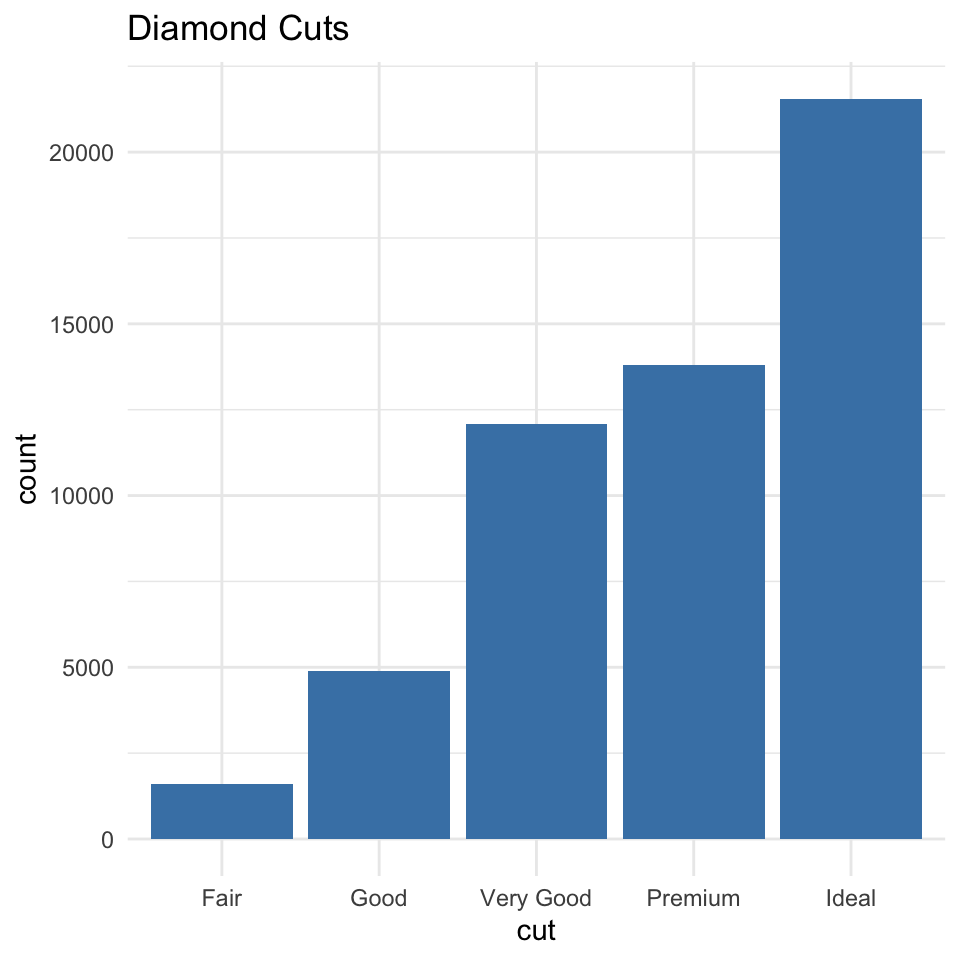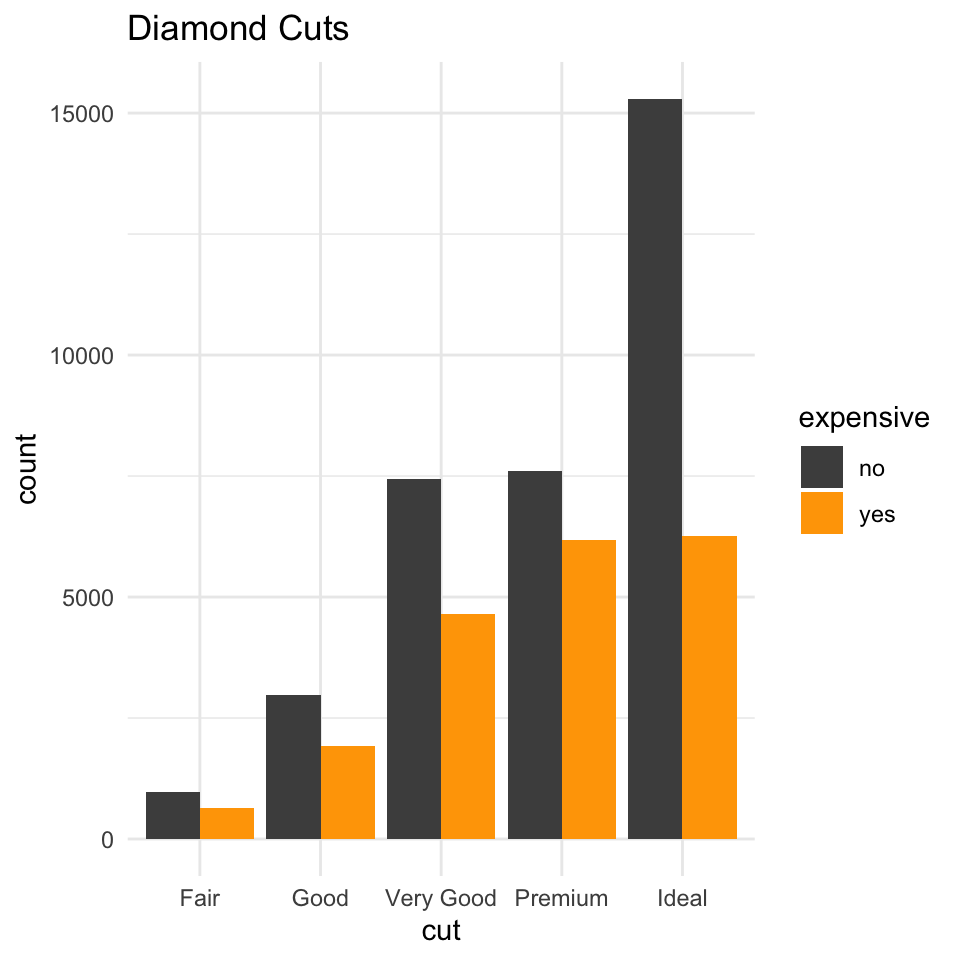# Learning Objectives

In the Asynchronous Lecture

• Understanding the components of a good quantitative visualization
• An introduction to the grammar of graphics (`ggplot2`)
• Delving into the different `geom`etric projections available to you when visualizing:
• continuous on continuous data;
• continuous on categorical data; and
• categorical on categorical data.
• Tweaking aesthetics with themes and scales.
• Combining many plots with `facet`ing and `patchwork`

In the Synchronous Lecture

• Further develop our understanding of the `ggplot2` package with an applied walkthrough
• Discuss how to export `ggplot2` graphics.
• Discover some third-party packages that make specific visualization tasks easier.

If you have any questions while watching the pre-recorded material, be sure to write them down and to bring them up during the synchronous portion of the lecture.

# Asynchronous Materials

The following tabs contain pre-recorded lecture materials for class this week. Please review these materials prior to the synchronous lecture.

Total time: Approx. 1 hour and 12 minutes

## Grammar of Graphics

### Code from the video

``````require(tidyverse)

# Data ------------------------------------------------------------------

# Simulated Data
set.seed(1234)
dat <-
tibble::tibble(var1 = rnorm(100),
var2 = var1 + rnorm(100))

summary(dat)

# Grammar of Graphics --------------------------------------------------
ggplot(data = dat, aes(x = var1, y = var2, color = var1)) +
geom_point()``````

## Continuous Data

### Code from the video

``````require(tidyverse)

# Data --------------------------------------------------------------------

# Simulated Data
set.seed(1234)
dat <-
tibble::tibble(var1 = rnorm(1000),
var2 = var1 + rnorm(1000))

summary(dat)

dat

# Continuous Univariate -----------------------------------------------------

# Histogram
ggplot(data = dat, aes(x = var1)) +
geom_histogram()

# Density Plot
ggplot(data = dat, aes(x = var1)) +
geom_density(fill="blue",color="white",alpha=.5)

# Continuous Bivariate ----------------------------------------------------

# Scatter Plot
ggplot(data = dat, aes(x = var1, y = var2)) +
geom_point()

# Line Plot
ggplot(data = dat, aes(x = var1, y = var2)) +
geom_line()

# Density Plot (in 2 Dimensions)
ggplot(data = dat, aes(x = var1, y = var2)) +
geom_density_2d()

# Same thing filled
ggplot(data = dat, aes(x = var1, y = var2)) +
geom_density_2d_filled()

# Hex Plot
ggplot(data = dat, aes(x = var1, y = var2)) +
geom_hex()

# Layering Geoms ----------------------------------------------------

ggplot(data = dat, aes(x = var1, y = var2)) +
geom_point() +
geom_hex(alpha=.7)``````

## Categorical Data

### Code from the video

``````require(tidyverse)
library(palmerpenguins) # https://github.com/allisonhorst/palmerpenguins

# Includes measurements for penguin species, island in Palmer Archipelago,
# size (flipper length, body mass, bill dimensions), and sex. This is a
# subset of penguins_raw.

# Data --------------------------------------------------------------------

dat <- penguins

summary(dat)

# Univariate Categorical --------------------------------------------------

# Bar Plot
ggplot(dat,aes(x=species)) +
geom_bar()

# Ordering Bar Plot by Frequency
ggplot(dat,aes(x=fct_infreq(species))) +
geom_bar()

# Adding in more categorical data
ggplot(dat,aes(x=fct_infreq(species), fill = sex)) +
geom_bar()

# Stacking vs. Dodge
ggplot(dat,aes(x=fct_infreq(species), fill = sex)) +
geom_bar(position="dodge")

# Bivariate: category on continuous -----------------------------------------

# Box plot
ggplot(dat,aes(x=body_mass_g,y = species)) +
geom_boxplot()

# Violin plot
ggplot(dat,aes(x=body_mass_g,y = species)) +
geom_violin()

# Jitter plot
ggplot(dat,aes(x=body_mass_g,y = species)) +
geom_jitter(height = .05,alpha=.5)

# Layer the representations
ggplot(dat,aes(x=body_mass_g,y = species)) +
geom_jitter(height = .15) +
geom_violin(alpha=.5)

# Trivariate: category on category on continous -----------------------------------------

dat %>%
drop_na() %>%
ggplot(aes(x=sex,y = species,fill=body_mass_g)) +
geom_tile()

# Trivariate: category on category on continous -----------------------------------------

dat %>%
ggplot(aes(x=bill_length_mm,
y = flipper_length_mm,
color=species)) +
geom_point()``````

## Many Plots

### Code from the video

``````require(tidyverse)
require(patchwork) # for combining plots
require(palmerpenguins) # https://github.com/allisonhorst/palmerpenguins

# Includes measurements for penguin species, island in Palmer Archipelago,
# size (flipper length, body mass, bill dimensions), and sex. This is a
# subset of penguins_raw.

# Data --------------------------------------------------------------------

dat <- penguins

summary(dat)

# Many Plots with Faceting -----------------------------------------

ggplot(dat,
aes(x=bill_length_mm,
y = flipper_length_mm,
color=species)) +
geom_point()

# Break plots up by category with facets
ggplot(dat,
aes(x=bill_length_mm,
y = flipper_length_mm,
color=species)) +
geom_point() +
facet_wrap(~species)

# Adjust scales on the facets
ggplot(dat,
aes(x=bill_length_mm,
y = flipper_length_mm,
color=species)) +
geom_point() +
facet_wrap(~species,scales = "free")

# Facet along more than one category
ggplot(drop_na(dat),
aes(x=bill_length_mm,
y = flipper_length_mm,
color=species)) +
geom_point() +
facet_wrap(~species + sex)

# Specify the columns and rows
ggplot(drop_na(dat),
aes(x=bill_length_mm,
y = flipper_length_mm,
color=species)) +
geom_point() +
facet_wrap(~species + sex,scales = "free",ncol = 2)

# Combine Plots with Patchwork --------------------------------------------

plt1 <-
ggplot(dat,aes(x=bill_length_mm,
y=flipper_length_mm)) +
geom_point()
plt1

plt2 <-
ggplot(dat,aes(x=fct_infreq(species), fill = sex)) +
geom_bar(position="dodge")
plt2

# Combine them with patchwork
plt1 + plt2

# Arrange them
plt1 + plt2 + plot_layout(ncol = 1)

# resize them
plt1 + plt2 + plot_layout(ncol = 1,heights = c(.25,.75))

# sky is the limit
plt1 + plt2 + plt2 + plt1 ``````

## Customizing Plots

### Code from the video

``````require(tidyverse)
require(palmerpenguins) # https://github.com/allisonhorst/palmerpenguins

# Includes measurements for penguin species, island in Palmer Archipelago,
# size (flipper length, body mass, bill dimensions), and sex. This is a
# subset of penguins_raw.

# Data --------------------------------------------------------------------

dat <- penguins

summary(dat)

# Customizing Plot Aesthetics -----------------------------------------

ggplot(dat,
aes(x=bill_length_mm,
y = flipper_length_mm,
color=species)) +
geom_point(size=3,alpha=.75) +
scale_color_manual(values = c("darkred","steelblue","grey30")) +
theme_classic() +
labs(x = "Bill Length (mm)",y="Flipper Length (mm)",color="") +
theme(legend.position = "top")``````

# Practice

These exercises are designed to help you reinforce your grasp of the concepts covered in the asynchronous lecture material.

For the following questions, we’ll use the `diamonds` dataset, which comes installed when we import the `ggplot2` package. The dataset contains the prices and other attributes of 54,000 diamonds. The dataset is useful for visualization purposes.

``````require(tidyverse)
dat <- diamonds
``````## # A tibble: 6 x 10
##   carat cut       color clarity depth table price     x     y     z
##   <dbl> <ord>     <ord> <ord>   <dbl> <dbl> <int> <dbl> <dbl> <dbl>
## 1 0.23  Ideal     E     SI2      61.5    55   326  3.95  3.98  2.43
## 2 0.21  Premium   E     SI1      59.8    61   326  3.89  3.84  2.31
## 3 0.23  Good      E     VS1      56.9    65   327  4.05  4.07  2.31
## 4 0.290 Premium   I     VS2      62.4    58   334  4.2   4.23  2.63
## 5 0.31  Good      J     SI2      63.3    58   335  4.34  4.35  2.75
## 6 0.24  Very Good J     VVS2     62.8    57   336  3.94  3.96  2.48``````

## Question 1

Using the `diamonds` data, plot the distribution of the different `cut` categories. Customize the plot as follows:

• Change the `fill` of the bars be `"steelblue"`;
• Change the theme of plot to be `minimal`;
• Add a title using `labs()` that reads “Diamond Cuts”

### _

``````ggplot(dat,aes(x=cut)) +
geom_bar(fill='steelblue') +
labs(title = "Diamond Cuts") +
theme_minimal()``````## Question 2

Do the following:

• Create a new variable in the `diamonds` data called `expensive` that takes on the value of `"yes"` if the price is above the average price of a diamond, `"no"` otherwise.
• Using the same code you used to generate the plot in Q1, use this variable to break up each category into expensive and non-expensive stacks using the `"dodge"` position (see the video on categorical data).
• When `expensive == "yes"` the bars should be filled `orange`, and `grey30` otherwise.

### _

``````dat %>%
mutate(expensive = ifelse(price > mean(price),"yes","no")) %>%
ggplot(aes(x=cut,fill=expensive)) +
geom_bar(position="dodge") +
scale_fill_manual(values = c("grey30","orange")) +
labs(title = "Diamond Cuts") +
theme_minimal()``````## Question 3

Using the `diamonds` data, plot `price` on `carat` using points, and do the following:

• Make the points transparent using `alpha = .25`;
• Color each point by `cut`.
• Facet the plots by `cut` and arrange the facet plots so that you only have 1 row;
• Change the labels so that the legend reads “Diamond Cut”, the x-axis reads “Price (\$)” and the y-axis reads “Carat”.
• Change the theme to `classic`;
• Change the position of the legend so that it’s on the `"bottom"` of the plot.

### _

``````dat %>%